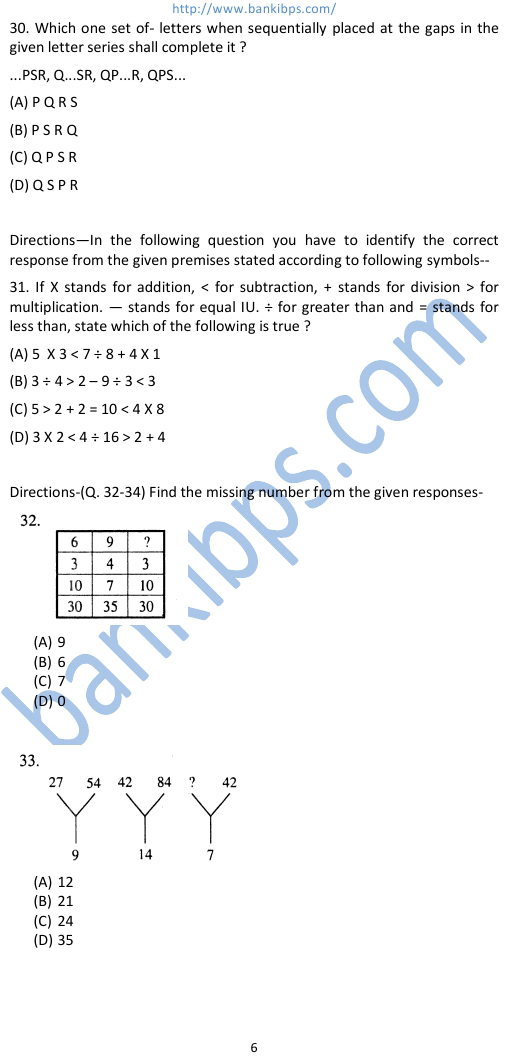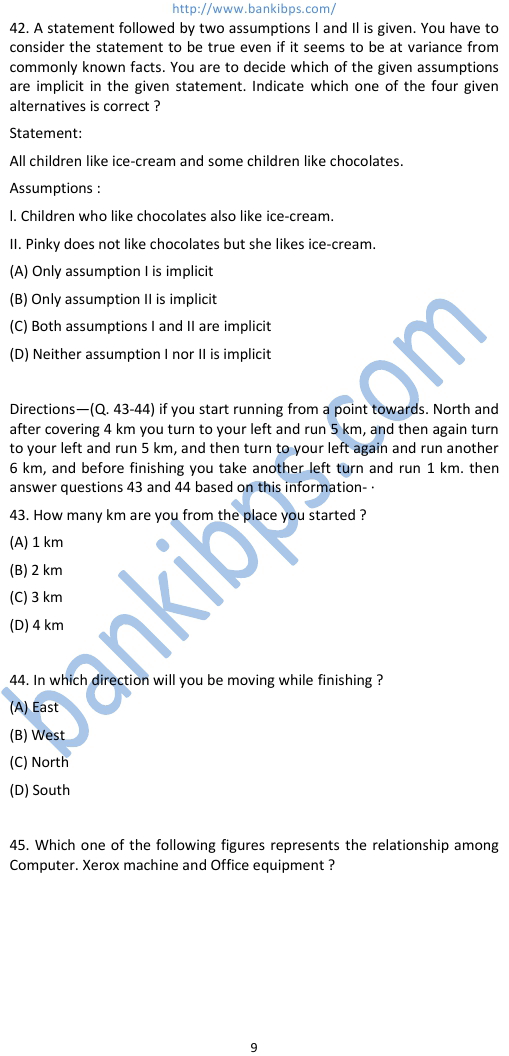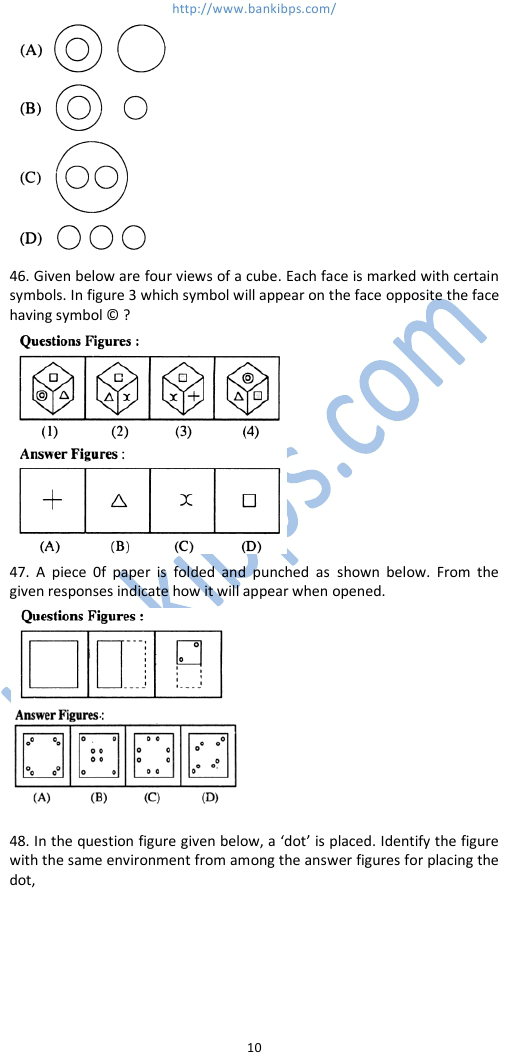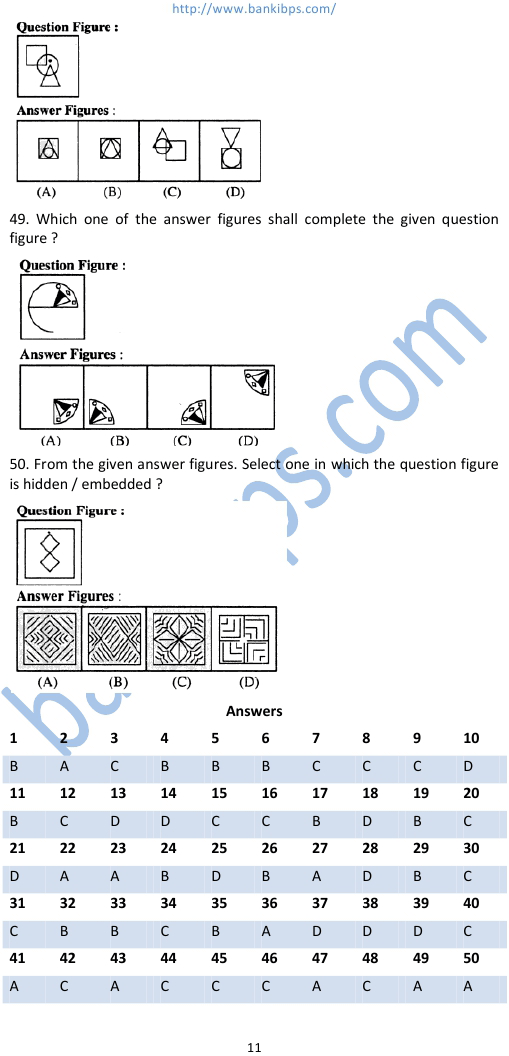# SSC Combined Graduate Level Exam Sample Question Papers

30. Which one set of- letters when sequentially placed at the gaps in the given letter series shall complete it ? ...PSR, Q...SR, QP...R, QPS... (A) P Q R S (B) P S R Q (C) Q P S R (D) Q S P R Directions—In the following question you have to identify the correct response from the given premises stated according to ssc combined graduate level exam sample question papers following symbols-- 31. If X stands for addition, < for subtraction, + stands for division > for multiplication. — stands for equal IU. ÷ for greater than and = stands for less than, state which of the following is true ?